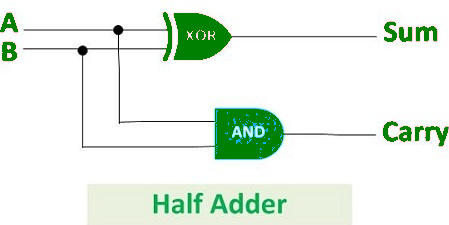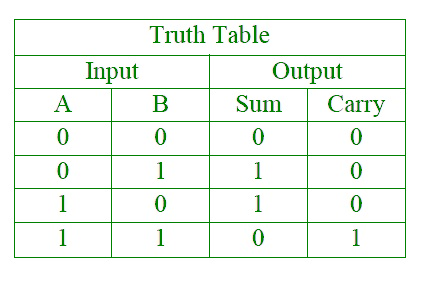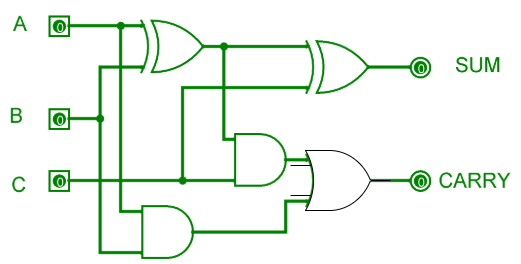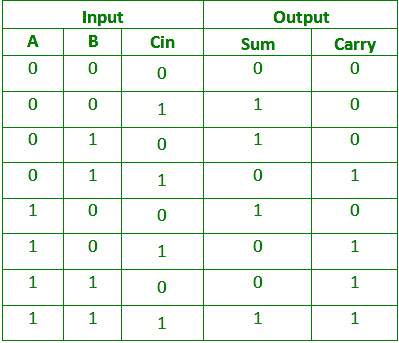Open In App

1. Half Adder : Half Adder is a combinational logic circuit which is designed by connecting one EX-OR gate and one AND gate. The half adder circuit has two inputs: A and B, which add two input digits and generates a carry and a sum.The output obtained from the EX-OR gate is the sum of the two numbers while that obtained by AND gate is the carry. There will be no forwarding of carry addition because there is no logic gate to process that. Thus, this is called the Half Adder circuit.

Logical Expression :

```Sum = A XOR B
Carry = A AND B ```

Truth Table :2. Full Adder: Full Adder is the circuit that consists of two EX-OR gates, two AND gates, and one OR gate. Full Adder is the adder that adds three inputs and produces two outputs which consist of two EX-OR gates, two AND gates, and one OR gate. The first two inputs are A and B and the third input is an input carry as C-IN. The output carry is designated as C-OUT and the normal output is designated as S which is SUM.The equation obtained by the EX-OR gate is the sum of the binary digits. While the output obtained by AND gate is the carry obtained by addition.

Truth Table:Logical Expression :

```SUM = (A XOR B) XOR Cin = (A ⊕ B) ⊕ Cin
CARRY-OUT = A AND B OR Cin(A XOR B) = A.B + Cin(A ⊕ B) ```